In this lesson, we will be learning about what lenses are and how they affect the path of light rays. Understanding lenses will be crucial in understanding how the eyes produce vision as well as how glasses work.

To understand the concepts covered in this lesson, it is best to have read:

Now let’s begin!

## What is a lens?

A piece of glass or other transparent substance with curved sides for concentrating or dispersing light rays.

The above definition touches upon the 2 critical properties of lenses:

1. They must be transparent
2. They must be curved

If either one of these criteria are not met, you do not have a lens.

#### Would a window be considered a lens?No. Although windows meets the first criterion of being transparent, they are flat (meaning they have no curvature) therefore they are not lenses.

#### Would a cereal bowl be considered a lens?No. Although cereal bowls are curved, they are not transparent (light does not pass through them) therefore they are not lenses.

#### Would a magnifying glass be a considered a lens?Yes! A magnifying glasses is transparent and its surfaces are curved therefore it acts as a lens.

Of course, a magnifying glass is just one example of one type of lenses. Lenses come in all different shapes and sizes and they are used in countless different applications.

## Lenses in Ray Diagrams

In Lesson 1 we learned what light rays and ray diagrams are. Now let’s add a lens into a ray diagram and see how it affects light rays.

First, let’s reiterate the 2 key properties of lenses and see what happens if either of them is missing.

Here is a ray diagram showing passing through a flat ‘lens’.Here is a ray diagram showing what happens to light attempting to pass through an opaque ‘lens’.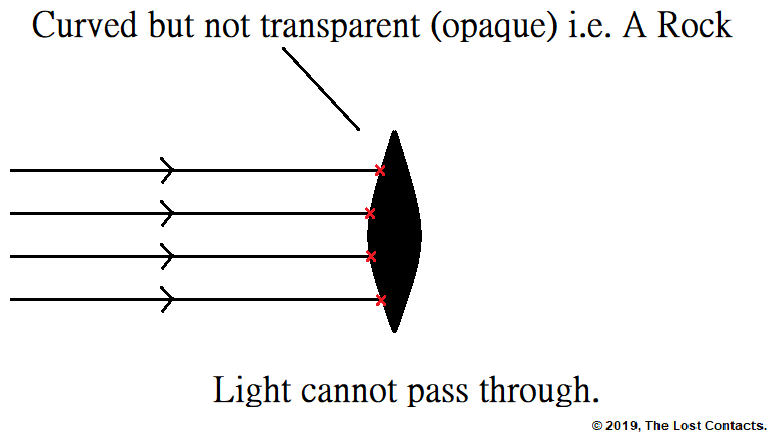Now if we have a proper lens, one that is transparent and curved, and we pass some light rays through it, the lens will affect the path of the light rays.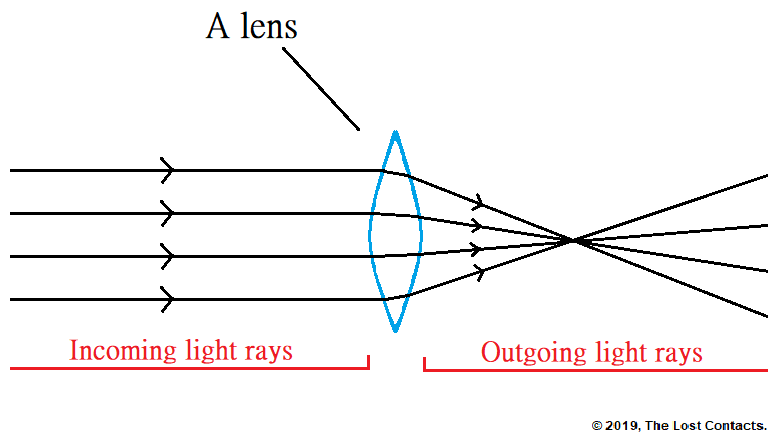As you can see from the example, the lens causes the light rays to change directions.

Of course, not all lenses have the same effect of light. There are sorts of different lenses that affect light in many different ways. For the rest of this lesson, we will focus mainly on the types of lenses used in glasses.

They are:

# Concave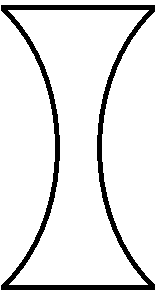An easy way to remember which is which: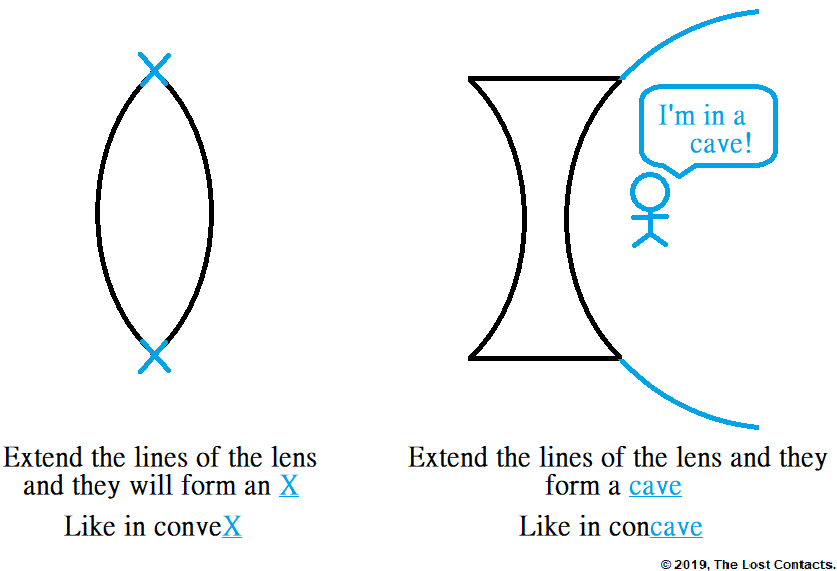Now let’s explore how each type of lens affects incoming light rays.

Until more advanced lessons, for simplicity, we will always consider incoming light that is parallel.

## Convex Lenses

Convex lenses add vergence to light that passes through them.

If you’re not familiar with what light vergence is, please review Lesson 1.

Recall that parallel light has zero vergence. So if you take the light with zero vergence and you add vergence to it, the result is light with positive vergence.

Also, recall that light with positive vergence is called converging light.

Therefore, the result of passing parallel light through a convex lens is converging light.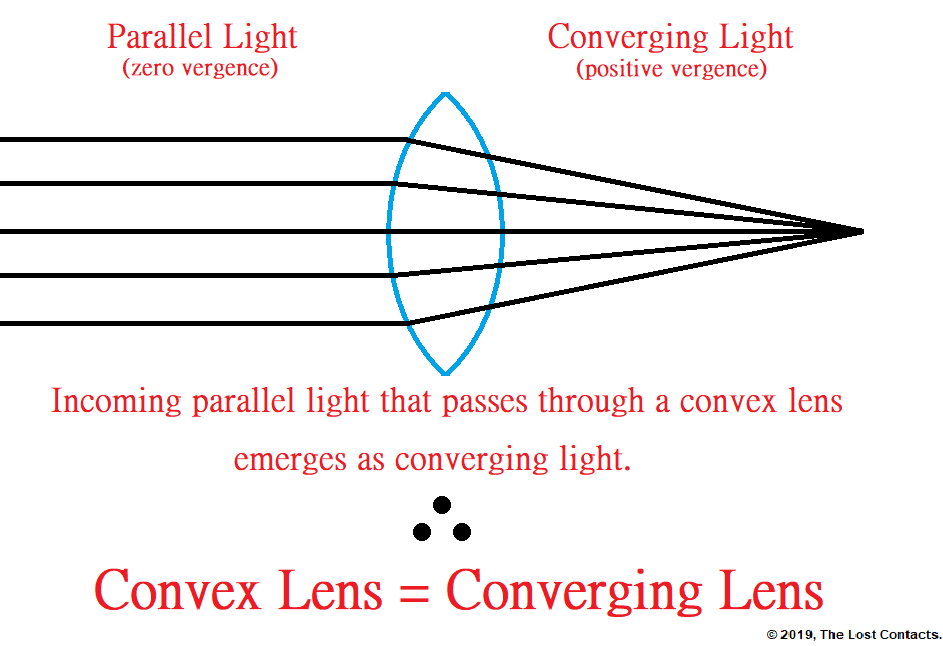Yes, another name for a convex lens is a converging lens. They mean the exact same thing. But if that wasn’t enough, there’s also another name for them!

Because convex/converging lenses increase the vergence of light, they are also called + (plus) lenses.

## Concave Lenses

Convex lenses decrease the vergence of light that passes through them.

Again, recall that parallel light has zero vergence. So if you take the light with zero vergence and you decrease its vergence, the result is light with negative vergence.

Also, recall that light with negative vergence is called diverging light.

Therefore, the result of passing parallel light through a concave lens is diverging light.Now you know that concave lenses are also known as diverging lenses because they cause parallel light to diverge. Another way looking at it is that concave lenses decrease the vergence of light. Because of this, they are also known as – (minus) lenses.

## Summary

Lenses must be made of transparent materials and must have a curved surface. Being transparent allows light to pass through them, and the curved surfaces cause the light passing through to change direction.

Glasses primarily use two different types of lenses. They are convex and concave.

Convex lenses cause parallel light passing through them to become convergent and concave lenses cause parallel light passing through them to become divergent.

Though the terms convex/concave and converging/diverging are useful in understanding the lenses, in the day-to-day operations of an optical shop, eye doctor clinic, lens manufacturing lab, etc., they are referred to simply with a + (convex/converging) or (concave/diverging) sign.

Now that we are familiar with the two main types of lenses, we will begin to explore the concept of lens power.